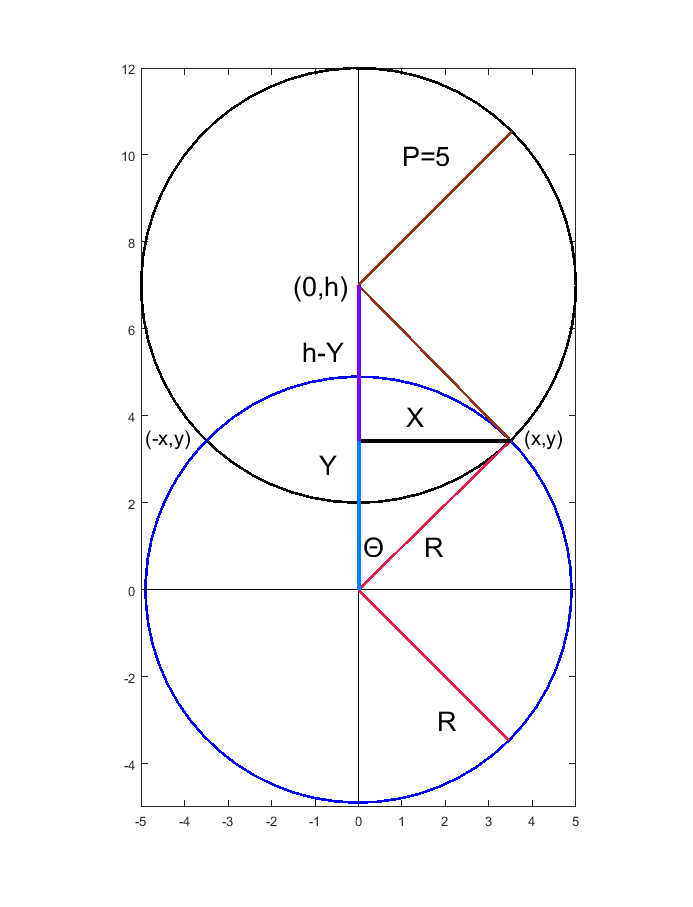# Problem 58862. Given Hypotenuse points create two right triangles

Given two points defining a hypotenuse create two right triangles of (h,5,R). Return the two (x,y) points that create the right triangles. I will elaborate on two geometric methods utilizing Matlab specific functions, rotation matrix, and translation matrix.
Given points [x1,y1] and [x2,y2] return [x3 y3;x4 y4] such that distance(xy2,xy3)=distance(xy2,xy4)=5. h>5
The below figure is created based upon h=distance([x1,y1],[x2,y2]), translating (x1,y1) to (0,0), and rotating (x2,y2) to be on the Y-axis. From this manipulation two right triangles are apparent: [X,Y,R] and [X,h-Y,5] with R^2+5^2=h^2. Subtracting and simplifying these triangles leads to Y and two X values after substituting back into R^2=X^+Y^2 equation.
P^2=X^2+(h-Y)^2 and R^2=X^2+Y^2 after subtraction gives R^2-P^2=Y^2-(d-Y)^2 = Y^2-d^2+2dY-Y^2=2dY-d^2 thus
Y=(R^2-P^2+h^2)/(2h) and X=+/- (R^2-Y^2)^.5
The trick is to now un-rotate and translate this solution matrix using t=atan2(dx,dy), [cos(t) -sin(t);sin(t) cos(t)] and [x1 y1]
A second method to find (X,Y) is theta=atan(5/R), X=Rsin(theta) and Y=Rcos(theta). The rotation and translation matrices are still required to return to the original coordinate system.In this figure h represents distance from (x1,y1) to (x2,y2) and (x1,y1) has been translated to 0,0

### Solution Stats

100.0% Correct | 0.0% Incorrect
Last Solution submitted on Aug 28, 2023

### Community Treasure Hunt

Find the treasures in MATLAB Central and discover how the community can help you!

Start Hunting!